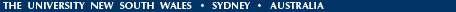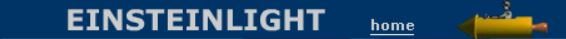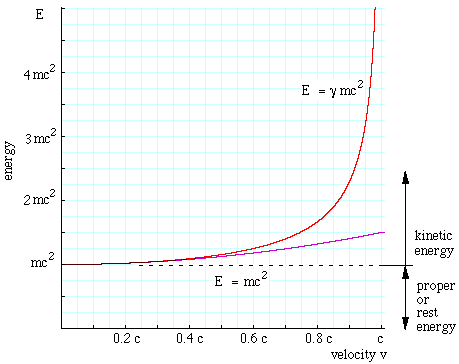Relativity in brief... or in detail..

E = mc2: What does it mean, and where did the equation come from?

E = mc2 is a famous, important and rather simple looking equation. It's natural to wonder what it means and where it comes from. We do that more formally in Energy in Newtonian mechanics and in relativity, but there we use mathematics. In this page, we try to explain that, with the absolute minimum of equations. But there have to be a few — we started with one!

What is work? and what is kinetic energy?

Lift a heavy object, and you'll know you've done work. Lift an object that is twice as heavy and you've done twice as much work*. Lift it twice as far, and you've done twice as much work. We define the work that you do on the object as the force you apply times the distance over which you move it. In shorthand, work is force times distance. (In this case, you can, in principle, get the work back: you could attach a rope to the now elevated objects, pass the rope over a pulley, and then lower the original objects to raise new objects. We say in this case that it is stored as gravitational potential energy.)
* A couple of complications. Here, if you lifted the object slowly, the force you applied was approximately equal to its weight. To move an object upwards, you need to apply a force a little more than its weight, to get it moving upwards, then a little less than its weight as it slows down at the top of the lift. Also, when you lift an object, you usually also lift your hand and other parts of your body. We're not including the work you do on yourself in this explanation.
When you throw a ball, you apply a nett force to it, and it accelerates from rest (before the throw) to some speed v when it leaves your hand. (v might be tens of metres per second.) Your hand moves in performing the throw, so you've done work on the ball. (More about acceleration in An introduction to the mechanics of Galileo and Newton.) In the film clip in Module 5, a spring provided the work that was converted to kinetic energy.
Acceleration of a mass using the force provided by a spring. How does that work? The horizontal bar here is an air track. Its hollow interior is supplied with high pressure air, which leaks out of tiny holes in the upper surfaces of the track. This turns the object above it into something like a miniature hovercraft. This almost removes friction. If only Aristotle had had one of those....

Let's consider a simple case, in which we apply a constant force over a certain distance to accelerate a mass, which we call m. You did work to accelerate that object. Where has the work gone? Once the object is moving, the work you did is converted into its kinetic energy.

It's easy to see that the kinetic energy is proportional to the mass: if you accelerate two similar objects (each mass m) to the same speed, you will have to do twice as much work. The kinetic energy increases with speed v, but it is not simply proportional — it is proportional to the speed squared. An example is well known to car drivers: if you double the speed, the braking distance is four times longer. A car travelling at twice the speed has four times as much kinetic energy, and so the same braking force (between the tires and the road) takes four times the distance to stop the car. (In this case, the kinetic energy is converted mainly into heat in the brakes, so four times as much heat is produced.) In fact, the kinetic energy is ½mv2.

Why v2? To accelerate it to higher v, you have to push it over a longer distance. We are keeping the force constant, so the acceleration, or change in speed per unit time, will be constant. So, to double the speed, we have to push it with the same force over twice the time. Now the average speed of an object subject to constant acceleration is half way between its initial speed (zero) and its final speed v, so the average speed is v/2. (This explains the "1/2" in the expression for kinetic energy.)

When we apply the same force for twice the time, its final speed and its average speed will both be doubled. So, travelling for twice as long at twice the average speed, it covers four times the distance during the acceleration, so we have to apply the force over four times the distance, so we do four times the work and it has four times the kinetic energy. (By the way, what we have just done is an informal version of the work-energy theorem, which shows that Newton's second law may be written in terms of energy, rather than force.)Why is relativistic kinetic energy different?

In Module 4, we saw that times and distances measured by observers in relative motion are different. So, different observers will also calculate different accelerations, different final speeds and different durations of the accelerations. To say much more than this requires mathematics, which we show in Energy in Newtonian mechanics and in relativity. For this page, where we are keeping equations to a minimum, let's just say that we get a different answer, as the graph shows.

However, several important questions remain. One is "what happened to the (½)? Why does kinetic energy in relativity have mc2 whereas classical kinetic energy has ½mv2?" The short answer to this is that the relativistic expressions involve the expression γ that depends on v. However, one thing that we do know is that Newtonian mechanics works very well at speeds much less than c, so we expect that, at low speeds, Einstein's and Newton's expressions must agree. In Energy in Newtonian mechanics and in relativity, we show explicitly that this is the case. In the graph, the purple line shows the classical kinetic energy, and the red line the relativistic kinetic energy.

Another is "how do relativistic and classical mechanics differ in practice?". The short answer is that, at ordinary speeds, the difference is almost zero. However, they become increasingly different as the speed gets closer to c. This is shown both in the graph and in the animation below, which is taken from Module 5. In this animation, we add energy to the space ship at a constant rate (which is not normal for rockets, by the way). In classical mechanics, the speed is proportional to the square root of the kinetic energy. But in relativistic mechanics, the kinetic energy is a stronger function of v (it varies more as v changes), so, at high speed, v has to rise only a little as the energy increases.

In fact, as v approaches c, the kinetic energy goes to infinity. This means that the rise in v gets smaller as we approach c: no matter how much energy we put in, the speed never gets to c.

Where does E = mc2 come from?

Remember that, in Module 4, we saw that clocks run slow and rulers appear short by a factor that depends on speed. That factor is called γ, and we shall write it here as γ(v) to show explicitly that it depends on v. A graph of γ(v) is plotted above. (Incidentally, in module 4, Jasper observed that the light followed the hypotenuse of a triangle, and γ comes from Pythagoras' law, which is why it has a square root. Details here).

We know that, at low speeds, clocks do not run slow and rulers don't shrink, so we know that, for v=0, γ=1. We could write this as γ(0)=1. When we do the maths, the kinetic energy in relativity turns out to be mc2-1), which we can write like this:

Kinetic energy = mc2γ(v) - mc2γ(0))
or, to write it without Greek letters:
Kinetic energy = mc2(correction factor for v) - mc2(correction factor when v = 0).
Now what do these terms mean? Just from its units, we know that mc2 is an energy. In classical mechanics, ½mv2 was an energy, so a mass multiplied by the square of a speed is an energy. So both terms are energy terms. We are used to seeing proper time and proper length multiplied or divided by this correction factor. Einstein's insight was to see that mc2 was the proper energy of mass m, the energy associated with mass m, independent of its motion. When it had relative speed, its energy was increased from γ(0)mc2 to γ(v)mc2, the difference between them being its kinetic energy.

Now you can get back the kinetic energy of a body by slowing it down to zero speed. For instance, you could attach a spring to it and let it stretch the spring until the body came to rest. In our example of the (ordinary) car, this energy is wasted as heat in the brakes, but in an electric train (or a well designed car) the kinetic energy is turned back into electrical power as the vehicle slows.

How would you obtain the mc2 energy? How would you obtain the proper energy of an object that it is already stationary? As this is associated with its mass, you must get it by reducing the mass.  Oversimplifying a little, we could say that, if you destroy a mass m you get an energy mc2. For example, when a uranium nucleus undergoes fission, the combined mass of the remnants is very slightly less than that of the original nucleus. So the energy produced in nuclear fission, whether under control in a nuclear power station, or explosive in a nuclear bomb can be related to a slight change in the masses of the nuclear particles involved.

The elements of medium atomic mass are the most stable. A large nucleus like uranium or plutonium can break up into less massive components, yielding fission energy. On the other hand, when two very small nuclei fuse together (e.g. two deuterium fusing to form helium), the helium is more stable and less massive. Again, the loss of mass m is associated with the energy mc2 released. Nuclear fusion may one day be the source of power in nuclear fusion reactors, whose advantages over fission reactors include the fact that helium is an innocuous gas (you can even sell it) and that the deuterium (or heavy hydrogen) comes from sea water, a small fraction of which is deuterated or heavy water.

The fact that the helium atom has less mass than that of the two deuterium atoms that fuse to produce it tells us that it takes energy to 'pull' the deuterium nuclei apart. We have to do work against the attractive forces between deuterium nuclei, and that work appears as a greater mass in the unfused nuclei. (For more about such forces, see binding energy.)

I am in danger here of giving the impression that this quantification of energy conversion is only important in nuclear reactions. Not true: it is just as applicable to ordinary chemical reactions as well. In chemistry, however, the energies involved are so much smaller that the change in mass is difficult to measure. In principle, we would expect it to apply wherever work is done: when you do work W on an object by lifting it, one would expect its mass to increase by W/c2. However, because c is so large, this mass would be much smaller than other effects (e.g. the mass of your fingerprints) and would be impossible to measure. To see some values, follow this link.

Richard Feynman on explanations with and without mathematics

The explanation I have given here may sound less than totally convincing. The more mathematical version is more persuasive, because it uses the reasoning power of mathematics. Richard Feynman was a brilliant physicist. He was also a brilliant lecturer. If I have failed you with these explanations, perhaps he would not. However, when it comes to mathematics, he might have claimed the same extenuating circumstance. In a collection of popular lectures, he said:
"it is impossible to explain honestly the beauties of the laws of nature in a way that people can feel, without their having some deep understanding of mathematics. I am sorry, but this seems to be the case.

You might say, 'All right, then if there is no explanation of the law, at least tell me what the law is. Why not tell me in words instead of in symbols? Mathematics is just a language, and I want to be able to translate the language'. In fact I can, with patience, and I think I partly did. I could go a little further and explain in more detail that the equation means that if the distance is twice as far the force is one fourth as much, and so on. I could convert all the symbols into words. In other words I could be kind to the laymen as they all sit hopefully waiting for me to explain something. Different people get diferent reputations for their skill at explaining to the layman in layman's language these difficult and abstruse subjects. The layman searches for book after book in the hope that he will avoid the complexities which ultimately set in, even with the best expositor of this type. He finds as he reads a generally increasing confusion, one complicated statement after another, one difficult-to-understand thing after another, all apparently disconnected from one another. It becomes obscure, and he hopes that maybe in some other book there is some explanation. . . . The author almost made it - maybe another fellow will make it right.

But I do not think it is possible, because mathematics is not just another language. Mathematics is a language plus reasoning; it is like a language plus logic. Mathematics is a tool for reasoning."

From: Feynman, R.P. (1965) "The Character of Physical Law" BBC. Reprinted by Penguin Books, 1992.Home | Summary | Quiz | Credits School of Physics - The University of New South Wales Sydney, Australia 2052 © School of Physics UNSW 2052 Disclaimer feedback